# More Coupled Oscillators

Wed, Jan 19, 2022 tags: [ physics julia programming ]

A very important concept in condensed matter physics (or solid-state physics, specifically) are lattice vibrations and their quantized form, phonons. I’ve mentioned before that I like using a computational approach to better understand concepts, and it’s not different here.

I will assume no prior knowledge on your part, and additionally, I want to state that I don’t claim to be anything but a humble student of this matter. If you find errors, please let me know discretely to save me the embarrassment :)

This article will consist of a few examples of computing the dynamics of simplified lattices using existing differential equation solvers, of course in Julia – especially in a topic like this you can feel that the language and its ecosystem were made for this.

Besides, the title “More Coupled Oscillators” suggests I’ve written about this before. I haven’t – but I have implemented this concept multiple times before. Only now did I do it in a way that seems worth sharing.

## Harmonic Oscillators

If you’ve had physics lectures in university or advanced lessons in school, feel free to skip this.

I learned about harmonic oscillators in the first semester of my studies, as does every other physics student. I was promised that they would remain important over the course of the studies, and they did! So what is a harmonic oscillator?

Intuitively, it’s any system that responds with a linear force to a displacement. The most obvious example of this is a metal spring. Further systems are for example an air-filled buffer, mostly any elastic material (such as rubber bands), to some extent a swinging pendulum (as found in old clocks), but also some types of atomic bonds. Oftentimes, a system is only approximately a harmonic oscillator. You will see why this is very useful.

It’s called “oscillator” because, when left loose and without too much damping (e.g. by friction), it will oscillate, i.e. swing back and forth.

Mathematically, such a system can be described by a function $x(t)$ giving the displacement at time $t$. The linear response is described using a spring constant $k$ so that the response force $F$ is equal to $$F = -k x.$$

The sign tells us that the force is directed opposite the displacement.

As most systems in the natural world, the harmonic oscillator can be described using a differential equation. In fact, it can be described so nicely that it is often given as one of the first examples for differential equations in the physics curriculum. If you don’t know or have forgotten: A differential equation (DE) is an equation containing a function and one or more of its derivatives (if you don’t know what a derivative is, I suggest you quickly look it up). I kind of tricked you, though: the DE was already introduced! It was

$$F = -k x!$$

The derivative is hidden in the $F$, which according to Newton is equal to $F = m a$. The acceleration $a$ is the time derivative of the velocity $v = dx/dt = \dot x$: $$a = d^2 x/dt^2 = \ddot x.$$

Thus in the full form, we can see $$F = m \ddot x = - k x.$$

The most frequent problem with differential equations is that we don’t know the solution, that is, the function $x(t)$ fulfilling the equation. For the simple harmonic oscillator, we are lucky though: Functions that, twice differentiated, reproduces itself with a negative sign (and times a constant factor) are most prominently the trigonometric functions $\sin(\omega t), \cos(\omega t)$. They are said to solve the differential equation. Let $x(t) = A \sin(\omega t)$, then

$$m \ddot x = -m A \omega^2 \sin(\omega t) = - k A \sin(\omega t) = - k x$$

And because this is a linear differential equation (the function $x$ appears “plainly”, without any powers or other functions), sums of different solutions are valid solutions, too. A general form of the solution is therefore $$x(t) = A \sin(\omega t) + B \cos(\omega t).$$

The $\omega$ in this case is the angular frequency $\omega = 2\pi / T$ with the periodic time $T$. It specifies how “fast” the oscillator vibrates.

It’s nice knowing the solution to this problem, now. It also gives us the answer to the question: With which frequency does an oscillator of mass $m$ and spring constant $k$ oscillate? As seen with the sine/cosine functions, the DE can be written as $$\ddot x + \omega^2 x = 0.$$

Therefore, by rewriting the original form $m \ddot x + k x = 0 = \ddot x + \frac{k}{m} x$, we see that $\omega^2 = k/m$. I.e., the frequency of the spring oscillator is determined by the spring constant and its mass. (by the way: we assume here that the spring itself doesn’t have a mass – this makes the calculations a lot easier).

## Solving the Harmonic Oscillator

With the foundations laid, an important skill is to solve differential equations numerically. In the special case above, we’ve found an analytical solution that can be written in a closed form, but this is not always the case – especially for more complicated systems as shown later. As this approach for solving DEs is important for the rest of the article, take this simple example to see how to leverage an existing solver for a trivial harmonic oscillator.

The differential equation to be solved is $$\ddot x = -\frac{k}{m} x$$ where $x = 0$ is the equilibrium (rest) position, and the initial position is $x_0 = 1$.

using DifferentialEquations
using Plots

theme(:ggplot2)

# The differential equation.
function ho(du, u, p, t)
du = u
du = -p[:k]/p[:m] * u
end

# The initial state of the system: u is a vector containing [x, dx/dt].
# I.e.: Displaced by one unit, and no velocity initially.
u0 = [1, 0]
# Further parameter: spring constant and mass.
p = Dict(:k => 1, :m => 1)

# Initialize problem: Using DE, initial state, integration period (from t=0 for three full periods),
problem = ODEProblem(ho, u0, (0, 3*2pi/sqrt(p[:k]/p[:m])), p)

# Sounds easy, doesn't it?
solution = solve(problem)

# Plot time vs. position (time is the 0th variable here, by convention).
plot(solution, vars=(0, 1), label="x(t)", xlabel="t", ylabel="x")The solution is, as is visible in the figure above, a clean cosine oscillation. Why not a sine? This was determined by the initial condition $u_0 = [1, 0]$. Had the $t= 0$ position been 0 (and the velocity not equal to 0), the solution to the DE would be a sine.

This may be the second-easiest task to solve with the DifferentialEquations package. The only complication is formulating the second-order DE in terms of first-order equations: you see, the harmonic oscillator depends on the acceleration, which is the second derivative of the position. However, the ODEProblem solver only treats first-order differential equations. What gives? We separate the problem into two coupled equations, with two variables $x$ (as known) and $y = dx/dt$:

$$(1) ~~ \frac{dx}{dt} = y$$ $$(2) ~~ \frac{dy}{dt} = - \frac{k}{m} x$$

For the program, we write the two variables as a vector $\tilde x = [x, y]$. The above system works trivially, as you can see when substituting in (2): $\frac{dy}{dt} = \frac{d^2x}{dt^2} = -\frac{k}{m} x$, which is the original DE.

Come to think of it: this can be written as a matrix-vector product!

$$\frac{d\tilde x}{dt} = \begin{pmatrix} 0 & 1 \\ -\frac{k}{m} & 0 \end{pmatrix} \tilde x = \begin{pmatrix} 0 & 1 \\ -\frac{k}{m} & 0 \end{pmatrix}\begin{pmatrix}x \\ \frac{dx}{dt} \end{pmatrix}$$

(Remember that a matrix $A$ with elements $A_{ij}$ (row $i$, column $j$) is multiplied with a vector as $(A u)_i = \sum_j A_{ij} u_j$)

function ho1(du, u, p, t)
A = [      0        1;
-p[:k]/p[:m]   0]
du .= A * u
end


This is a bit overkill for now, and this way of coding is certainly the most inefficient way (allocating the same array for every function call), but you can see that it works and is the same as written above. Why this is a nice way to write is something that will become clear soon.

## Coupled Systems

An example that already showed up in theoretical physics lectures during the first semester of my studies is the problem of coupled mass oscillators, which can be imagined a little bit like this:

  /|   1          2          3  |\
/|-VVVV-[###]-VVVV-[###]-VVVV-|\
/|                            |\
|          |
x1 = 0     x2 = 0


Let me explain this sketch: Two walls on the left and right are connected to metal springs (VVVV), which in turn connect to masses [###]. The two masses are connected to each other as well. The following steps will describe in detail how to translate this system into a (system of) DE at first, and into a computationally tractable problem secondly.

The question now is: how do these masses swing? Initially they are assumed to be of mass $m$ and connected by springs with the spring constant $k$ (later we may use different masses instead of equal ones, or different spring constants).

To solve this, the equations of motion are needed. In the previous example, the equilibrium position was fixed to 0, and it makes sense to do the same here. However, we have two masses – which position will be the true zero? This doesn’t matter, in fact, because for the relative forces between them, only the displacement matters, i.e. the distance to each mass’ equilibrium position. Therefore, it is reasonable to set two points to be the zero point, one for each mass. This is indicated above. The relevant coordinates $x_1, x_2$ are therefore the displacements from the respective equilibrium position. The $x$ axis points to the right, which is important for the signs of the coordinates.

Considering the first oscillator $m_1$, what are the forces moving it?

• Once moved slightly to the left, the first mass will experience
• a force from the left spring attempting to push it back to the equilibrium. This depends only on $x_1$, as the left end of the first spring is fixed to a wall.
• a force from the right spring attempting to pull it back to the equilibrium. However, it becomes a bit more tricky here, because the other end of the second spring is not fixed, but hangs on the second mass. This is the coupling: the force on the first mass depends on the position of the second one! Specifically, it is the difference $x_1 - x_2$ governing the second spring’s elongation or compression. If both masses have the same displacement, the spring will not “feel” any displacement and be in the resting shape. If the second mass is moved to the left, the spring will be compressed, and so on.

Therefore, the force on the first mass is: $F_1 = m \ddot x_1 = - k ( x_1 + (x_1 - x_2) = -k (2 x_1 - x_2)$.

Analogously, the force on the second mass is $F_2 = m \ddot x_2 = -k ( (x_2 - x_1) + x_2 ) = -k (-x_1 + 2 x_2)$.

Translated into a numerically solvable function, this might look like the following (you will recognize most of the structure from the previous example):

using DifferentialEquations
using Plots
theme(:ggplot2)

function cho(du, u, p, t)
du = u
du = u
du = -p[:k]/p[:m] * (2*u - u)
du = -p[:k]/p[:m] * (-u + 2*u)
end

p = Dict(:k => 1., :m => 1.)
u0 = [1, 0, 0, 0]

problem = ODEProblem(cho, u0, (0, 20), p)
solution = solve(problem)

plot(solution, vars=[(0, 1), (0, 2)], xlabel="t", ylabel="x", label=["\$x_1(t)\$" "\$x_2(t)\$"])


Again in the cho function, you can see the separation of the two coupled differential equations of second order into four differential equations of first order. The rest is again done by the DE solver. Plotting the solution yields the folllowing, more interesting figures: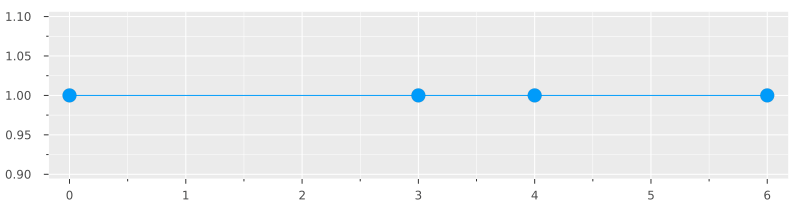For the animation, the equilibrium positions were chosen at $x_{0,1} = 2$ and $x_{0,2} = 4$, and the walls are set at 0 and 6. The initial displacement was $x_1 = 1$.

What we see here is a quite irregular-seeming pattern. What is visible is the transfer of energy between the oscillators, shown by the varying amplitudes of each point. One interesting question would be for those patterns of motion that are very regular, i.e. periodic, showing the same motion in one period as in the next.

To understand this, let’s go back to the matrix formulation. Here, this starts making more sense as there are already two equations invoked. We can translate the system of two equations above as such:

$$\begin{pmatrix}\ddot x_1 \\ \ddot x_2 \end{pmatrix} = -\frac{k}{m} \underbrace{\begin{pmatrix} 2 & -1 \\ -1 & 2 \end{pmatrix}}_{:= C} \begin{pmatrix} x_1 \\ x_2 \end{pmatrix}$$

For the purpose of numeric computation, this can be transformed into

$$\begin{pmatrix} \dot x_1 \\ \dot x_2 \\ \ddot x_1 \\ \ddot x_2 \end{pmatrix} = \begin{pmatrix} 0 & 0 & 1 & 0 \\ 0 & 0 & 0 & 1 \\ 2k/m & -k/m & 0 & 0 \\ -k/m & 2k/m & 0 & 0 \end{pmatrix} \begin{pmatrix} x_1 \\ x_2 \\ \dot x_1 \\ \dot x_2 \end{pmatrix}$$

If you hash out the matrix-vector multiplication, you will find the same in the code snippet above. Note that the matrix consists of two sub-blocks: The upper-right block links the velocity equations, whereas the bottom-left block defines the coupling. The bottom-right block, which is empty here, can be used to define damping (i.e., friction and other phenomena), which I’ll describe a bit further down. The upper-left block would allow linking velocity to position, which I don’t see a good reason for (let me know if there is!).

But let’s focus more on the first matrix for now. At first it seems to be a nice way of representing the calculation, but there is more meaning to be extracted from it. In fact, a meaning that was magic to me at the time when I first heard about it! Its secret lies in its so-called eigenvalues and eigenvectors. If you know what these are – great! If you don’t, here’s a quick-and-dirty summary: generally, a vector $u$ multiplied by a matrix $A$, $A u$ gives a new “transformed” vector. However, some matrices have a special effect on certain (matrix-specific) vectors: They don’t transform them just any way, but merely change their magnitude, i.e. length. These vectors are called eigenvectors (eigen coming from German for characteristic or inherent), and their scaling factors are the respective eigenvalues. This means that for an eigenvector $v$ with a scalar eigenvalue $\lambda \in \mathbb{R}$ of a matrix $A$, it holds that $A v = \lambda v$.

The eigenvectors and eigenvalues of a matrix can be calculated – I won’t describe this here –, especially in Julia using the LinearAlgebra.eigen function, for example. Alright: and now the magic! It turns out that if you calculate the eigenvalues of the matrix $C$ as defined above, the 2-dimensional eigenvectors will be those displacements that lead to periodic motion, and the respective eigenvalues are the squared angular frequencies $\omega^2$ of these so-called eigenmodes.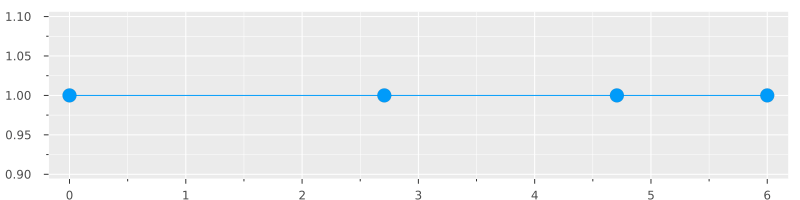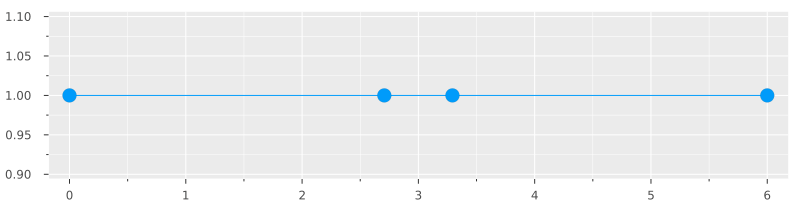The first eigenmode corresponds to the vector $v_1 = -\begin{pmatrix}\sqrt{2} \\ \sqrt{2}\end{pmatrix}$ with eigenfrequency $\omega_1^2 = \frac{k}{m}$, whereas the second one is $v_2 = \begin{pmatrix}-\sqrt{2} \\ \sqrt{2}\end{pmatrix}$, with eigenfrequency $\omega_2^2 = 3 \frac{k}{m}$. (Note that the magnitude of eigenvectors is scaled to 1 (unity), in order to be consistent with the eigenvalue.) As the problem is formulated in a way that each vector represents the displacements, you can see immediately that the first eigenmode has the two masses oscillating in synchrony, whereas the second one has them oscillating “against” each other, with a phaseshift of 180 degrees. The second mode also has a higher eigenfrequency, as you can imagine that the response to each oscillator is stronger if there is another one pushing or pulling against it at the point of highest displacement.

You will have noticed that the animations above seem to be swinging in harmony; this is only a result of the GIF creation. In truth, the second animation runs about 40% slower than the first, as the eigenfrequency of it is $\sqrt{3}$ times higher.

This mechanism doesn’t only apply for such simple systems here, but also more complicated ones as shown later on. In general, a system wiil have one eigenmode for each degree of freedom. Here, two masses can move in one direction (x), so that there are two eigenmodes in total.

Furthermore, all other oscillations can be described as superpositions of these two eigenmodes, further emphasizing the importance of the eigenvectors we found from the matrix above. And longitudinal oscillations as shown above are not the only way that a linear chain can swing. If an additional dimension is introduced, transversal oscillations are possible just as well. (and superposed combinations of both)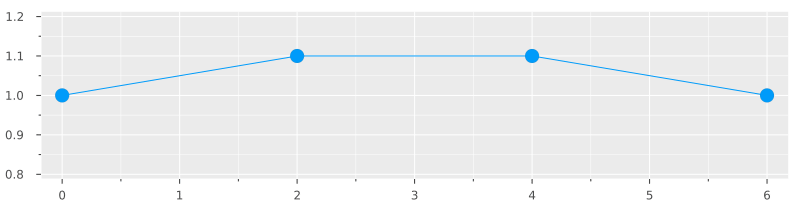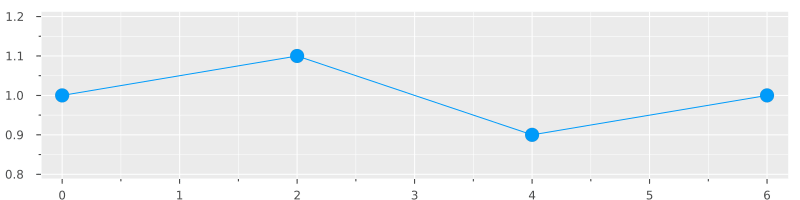Transversal eigenmodes: clearly analogous to the longitudinal ones.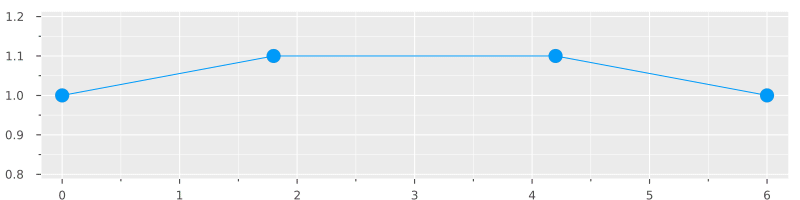Superposed oscillation of a longitudinal and a transversal eigenmode, and the respective trajectories.

This can be generalized to $n$ oscillators which are linked in the same way as described here. Instead of fixed ends, one might however use Born-von-Kármán boundary conditions, which effectively means arranging the oscillators in a ring and therefore foregoing special treatments of the first and last elements. This works especially well for large n. For completeness, here is the code for generating such a coupling matrix. As before, the odd elements are x coordinates and the even elements are y coordinates.

# The coupling matrix has 4nx4n elements for a linear chain of two degrees of freedom per oscillator.
# We use Born-von Karman boundary conditions, i.e. the chain is a ring.
function n_coupled_masses(n::Int, ks::Vector, masses::Vector; fixed_ends=false)
# Prepares coupling matrix.
if length(ks) != (n+1)
@show (length(ks), n+1)
error("Length of ks must be n+1.")
end
if length(masses) != n
@show n
error("Length of masses must be n.")
end

A = zeros(4n, 4n)
A[1:2n, 2n+1:4n] = LinearAlgebra.I(2n)

for i = 1:n  # oscillators
k1, k2 = ks[i], ks[i+1]

x = 2i-1
y = 2i

prevx = (x-2) < 1 ? (2n-1) : (x-2)
prevy = (y-2) < 1 ? (2n) : (y-2)
if !fixed_ends || ((x-2) >= 1)
A[2n+x, prevx] += k1/masses[i]
end
if !fixed_ends || ((y-2) >= 1)
A[2n+y, prevy] += k1/masses[i]
end

nextx = ((x+2)%(2n))
nexty = max(((y+2-1)%(2n)+1), 2)
if !fixed_ends || (nextx == x+2)
A[2n+x, nextx] += k2/masses[i]
end
if !fixed_ends || (nexty == y+2)
A[2n+y, nexty] += k2/masses[i]
end

A[2n+x, x] -= (k1+k2)/masses[i]
A[2n+y, y] -= (k1+k2)/masses[i]
end
A
end


It allows for specifying fixed ends or BvK boundary conditions. With fixed ends, a chain of $n = 20$ oscillators excited on the left would look like this: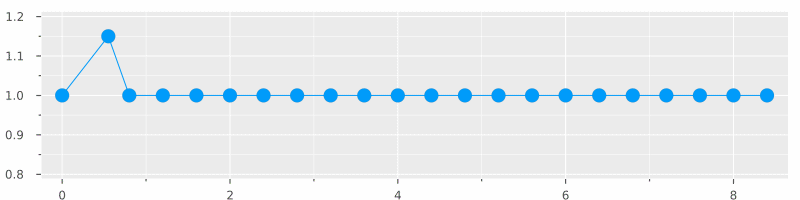By the way, a technicality: I have and will solve the equations of motion using the ODEProblem interface, even though there are dedicated interfaces in the DifferentialEquations library for this, especially the SecondOrderODEProblem type. However, I will keep sticking to the bottom-up approach here.

## Going two-dimensional

Linear chains are very important for demonstrating lattice dynamics, and generalize well to more complicated systems, such as certain crystal symmetries – there, you can model the behavior of a set of crystal planes as a linear chain.

However, it has obvious limits. Therefore it makes sense to look at a similar system but in two dimensions. I actually don’t plan on going three-dimensional in this post: it has the same principles but is more difficult to visualize and doesn’t bring much to the table unless we want to model an actual physical system quantitatively.

To create a two-dimensional oscillator system, we don’t have to do much more than for the linear chain, but the coupling matrix is slightly more complex now. Therefore, a little bit more code is required for generating it – see below. Also note that the rules are mostly made up: of course there needs to be next-neighbor coupling, but there is a bit of leeway in terms of coupling for example diagonal neighbors, or approximating nonlinear terms.

Another small new feature is the damping which can be emulated by adding terms in the bottom-right quadrant of the matrix. This is equivalent to the $\gamma$ terms in the friction version of the equation of motion: $$\ddot x + 2 \gamma \dot x + \frac{k}{m} x = 0$$ It depends on the velocity, therefore it must appear in the bottom-right quadrant.

function neighbors(n::Int, i::Int)::Tuple
# Returns neighbors in bottom/top/right/left
a = (i + n) % n^2
a = (a==0) ? n^2 : a

b = (i - n) < 1 ? (n*(n-1)+i) : (i-n)

c = i + 1 % n^2
c = (div(c-1, n) > div(i-1, n)) ? (i-n+1) : c

d = (i - 1) < 1 ? n : (i-1)
d = (div(d-1, n) < div(i-1, n)) ? (i+n-1) : d

(a,b,c,d)
end

function coupling_matrix(n::Int, k::Float64; damping::Float64=0., full=false)
N = n^2
if full
A = zeros(4N, 4N)
else
A = spzeros(4N, 4N)
end

A[1:2N, 2N+1:end] = LinearAlgebra.I(2N)

for i in 1:N
(b,t,r,l) = neighbors(n, i)
# Diagonal neighbors
(_, _, r1, l1) = neighbors(n, t)
(_, _, r2, l2) = neighbors(n, b)

x = 2i-1
y = 2i

# TO DO: Introduce non-linear coupling!

# Next-neighbor linear coupling
A[2N+x, 2r-1] += k
A[2N+x, 2l-1] += k
A[2N+x, 2i-1] -= 2k

A[2N+y, 2t] += k
A[2N+y, 2b] += k
A[2N+y, 2i] -= 2k

for e in (r1, r2, l1, l2)
A[2N+x, 2*e-1] += k/4
A[2N+x, 2*e]   += k/8 # Interaction between diag. neighbor y and this element's x is weaker.
A[2N+y, 2*e-1] += k/8
A[2N+y, 2*e]   += k/4
end
A[2N+x, x] -= 3/2 * k
A[2N+y, y] -= 3/2 * k

A[2N+1:end, 2N+1:end] -= LinearAlgebra.I(2N) * damping
end
A
end


The resulting coupling matrix can be used very easily now: as long as no anharmonic terms are required, one step is as easy as the following function, which will be used for the ODEProblem, where A is the coupling matrix:

function f2d(du, u, A, t)
LinearAlgebra.mul!(du, A, u)
end


What remains now is setting up the DE solver, just as before, and plotting the solution. A helper function makes it easy to set the initial state:

# This only allows for specifying initial displacement, not velocity.
function initial_state(n::Int, displacements::Vector{Tuple{Int, Int, Float64, Float64}})::Vector
u0 = zeros(4n^2)
N = n^2
for (x, y, dx, dy) in displacements
i = n*(x-1) + y
ix, iy = 2i-1, 2i
u0[ix] += dx
u0[iy] += dy
end
u0
end

# Nothing new here:

initial_xy_transversal_displacements = vcat(
[(1, i, 0.5, 0.0) for i in 1:n],
[(i, 1, 0.0, 0.5) for i in 1:n])

u0 = initial_state(n, initial_xy_transversal_displacements)
coupling = coupling_matrix(n, 1.; damping=0.0000, full=true)
problem = ODEProblem(f2d, u1, (0, 20), coupling)
solution = solve(problem)


A solution can be plotted: Here, in the first figure the left and bottom chains are initially displaced transversally. This leads to a jiggly rotation-like movement in the lattice. In the second figure, the displacements are longitudinal, showing a very different motion (obviously). Fascinatingly, the movements you see here are actually happening (more or less) in actual crystalline materials (ionic crystals and metals)! These oscillations are responsible for such diverse phenomena as heat capacity, heat conductivity, speed of sound, and even superconductivity (a state in which a material has no electrical resistivity – that is, not “just very small” but actually zero resistivity!). Those oscillations usually range between relatively low frequencies (for very long-wavelength excitations) to frequencies on the order of Terahertz (THz), i.e. $10^{12}$ oscillations per second. These vibrations can be quantized as phonons (compare to photons!), as mentioned in the introduction; phonons are quasi-particles representing units of oscillatory excitations in a solid body. By treating these phonons according to quantum mechanics and statistical physics, more accurate predictions can be made than using classical models. If you are interested, I recommend you read e.g. the Oxford Solid State Basics by Steven H. Simon – one of the best physics textbooks I’ve read so far.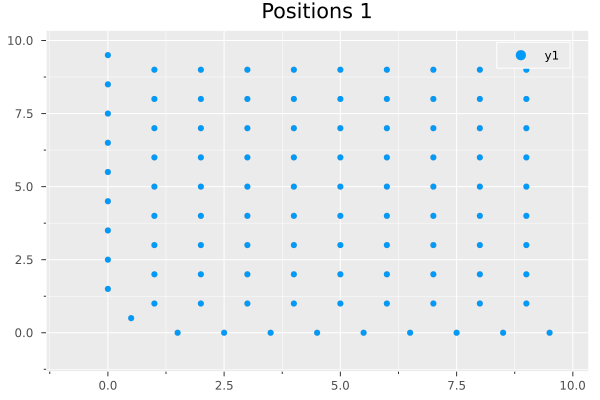Transversal oscillations in x-y direction.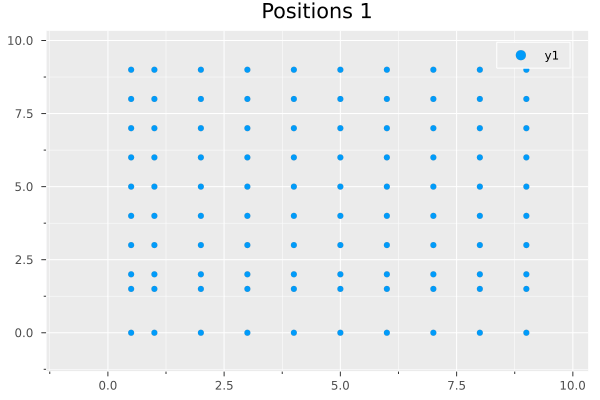Longitudinal oscillations in x-y direction. Note the superposed oscillations visible in the movement.

### Eigenmodes of the 2D lattice.

Before, I’ve already presented eigenmodes of the linear chain. It turns out that the very same mechanism applies for this two-dimensional system, for which we can just as well find the eigenvalues and -vectors by considering the coupling matrix. However, now there will be one eigenmode for each particle and each dimension – here, this will be $2N$ modes:

# Trick: only consider the coupling part of the DE matrix, i.e. the bottom-left quarter. So, just
# the same as before.
# Note that the matrix of eigenvectors is already (2N^2)x(2N^2), i.e. 200x200 for N=10 and 100
# oscillators.
ED = LinearAlgebra.eigen(coupling[2n^2+1:end, 1:2n^2]);

# Initialize a state from an eigenvector, padding the velocity part with zeroes.
# Just arbitrarily use the 32nd eigenmode.
u1 = vcat(ED.vectors[:,1] ./ 2, zeros(2n^2));


It turns out that the first resulting eigenstate using the numeric eigenvector computation is a longitudinal diatomic mode, where two lattice planes oscillate against each other: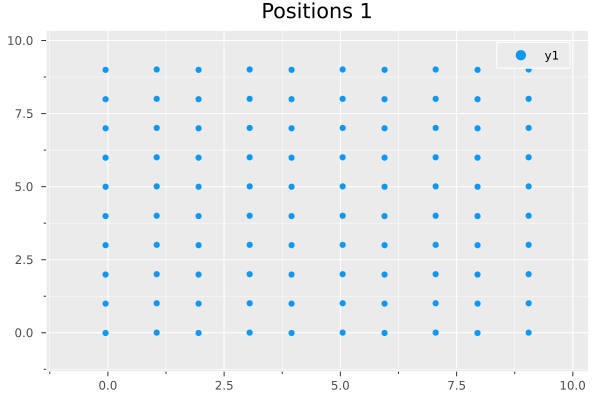The 150th eigenmode is already a lot more interesting. We see some particles almost frozen in place, whereas others move a lot around their position. This can be analyzed by showing the trajectories: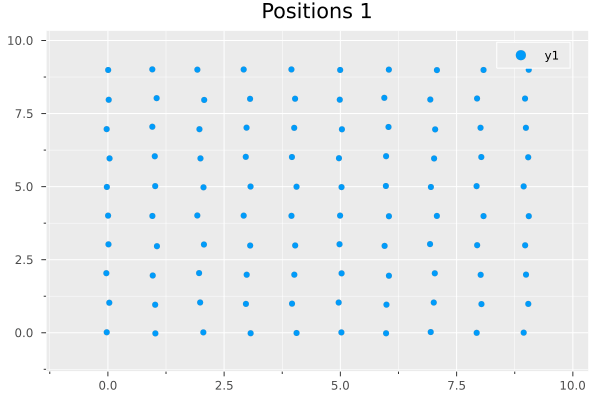Note that this is quite boring due to it being an eigenmode. If we go back to the longitudinal excitation from above (the second animation), the emerging trajectories are a lot more interesting to watch. Especially noteworthy are those oscillators moving in a straight line – showing the superposition of the different imposed excitations. Various figures are also found more than once, despite their seeming randomness! For example, the very top left oscillator’s movement is traced exactly by the one just above the bottom left corner. Further note that these trajectories are superpositions of some (or many) of the eigenmodes calculated above. It would certainly be interesting to decompose this movement into the eigenmodes; this should be possible using a Fourier transform (I guess?).### Interlude: Eigenmode decomposition

The (I guess?) irked me a bit – so I quickly used the lovely AbstractFFTs module to confirm my suspicion. I seem to have been correct: you can see this in the following pictures.

For a quick explanation of the following plots: The blue dots at $x=0$ are the calculated eigenfrequencies of the lattice as specified by the coupling matrix, and the orange line is the intensity, i.e. squared-absolute of the real-valued fast Fourier transform of the solution. It is clearly visible that eigenmodes are composed of one single frequency, whereas the mixed excitation is composed of at least six different eigenmodes! Due to the limited time interval, the peaks have a finite width, making it difficult to pin down to exactly one eigenmode (this was with $t \in [0, 50]$) – but you get the principle.

The Fourier transform was calculated using an arbitrary oscillator, here it was the x oscillation of the 75th point. Cross-checking (in the last of the three figures) reveals that the basic modes are the same for different oscillators and directions (x vs y) with slightly varying intensities.Eigenmode 1Eigenmode 150FFT of oscillations caused by initial longitudinal x-y displacement, for different oscillators in the lattice (different colors). I don’t know why the second peak is between eigenfrequencies – I possibly made a mistake plotting the eigenfrequencies?

This sort of plot is easily achieved using a few lines of code:

# Extract timeseries for nth oscillator (here n = 77)
xs = [solution.u[i] for i in 1:length(solution.u)]
# Apply FFT
rys = rfft(xs)
# Calculate frequencies
rxs = rfftfreq(length(solution.u), length(solution.u)/50.)

# Display eigenfrequencies, where ED is the result of LinearAlgebra.eigen().
scatter(abs.(ED.values)/(2pi), zeros(200), xlim=(0, 1), legend=false)
# Display Fourier transform (intensity).
plot!(rxs, abs.(rys).^2)


The maximum frequency is $\omega = 1 = \sqrt{k/m}$ with $m = k = 1$ in this case.